#### How to Use Forecast Function in Excel

In this lesson, you will learn how to use the forecast function and how to… predict the future. The Forecast function can either calculate or predict the future value with the help of the current value. A predicted value is the y-value that comes from a given x value. The popular values are both the x- and y-values, and the new value will be predicted with the help of linear regression.

This function is useful for predicting future sales, along with requirements for inventory, or even customer trends.

Thanks to the forecast function, Excel can read the future. It is not a joke! Excel can predict some values if you give it past data.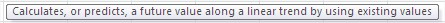You can use the Forecast function to predict simple data and also conduct advanced analysis for your company. Let’s see how the forecast function works and how to use it.

## Definition of FORECAST function

The forecast function has different arguments that are all required.

X: This is essential. It is a data point, for which the user wants to predict the value.

Known_y’s: This is also essential for the function. It is either a dependency array or data’s range.

Known_x’s: This is essential. An independent array that can also be a data range.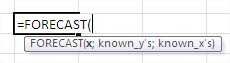It can look difficult, but it is not. Let’s see some examples to explain more.

## Forecast function examples

### How long do students study for the test?

You have some historical data about test equals. You also know, how long students have studied for the test.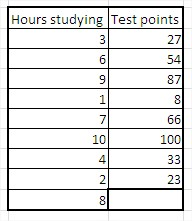You want to calculate how many points the student will get who has been studying for 8 hours. You can use the forecast function to calculate that.

x equals 8, because you are looking for a value for an 8-hour argument.

known y’s are test points, which you already know.

known x’s are hours of study, which you also know.

The formula in this situation is =FORECAST(B11,C3:C10,B3:B10) as shown in the picture below: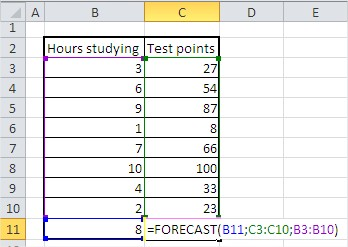The forecasted value is 77.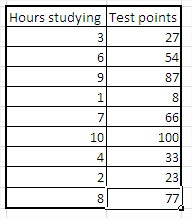Value 77 means that, based on historical values, the student will get 77 points. I hope the forecast function is easy and clear. Let’s look at one more example.

### Forecasting net income

You have sales and net income for some companies.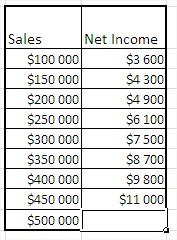You want to calculate the net income for a \$500,000 sale.

x is \$500,000

known y’s is historical net income.

known x’s are historical sales.

Formula here is =FORECAST(B11,C3:C10,B3:B10)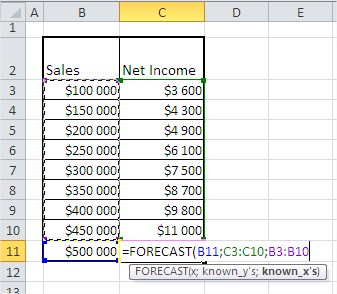Net income equals \$11,921.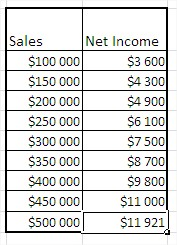### Date forecasting

You can also forecast dates. Here’s an example.

In this table, you have data on sales at the end of each month. You want to know where you will have a million dollars in sales.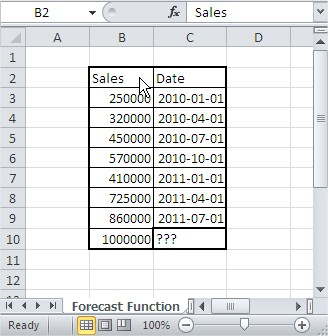x is \$1,000,000

known y’s are historical dates.

known x’s are historical sales.

Formula is: =FORECAST(B10;C3:C9;B3:B9)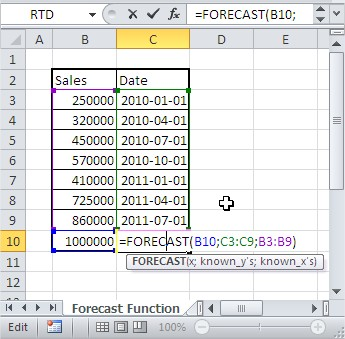The formatting of cells is General by default, so your result is not a date.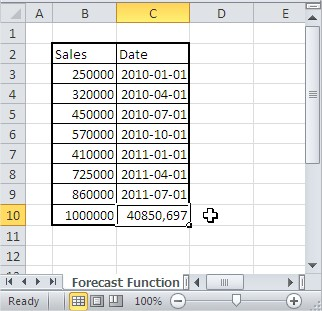Change the formatting of the cell. Click right and next Format Cells… and change formatting to Date.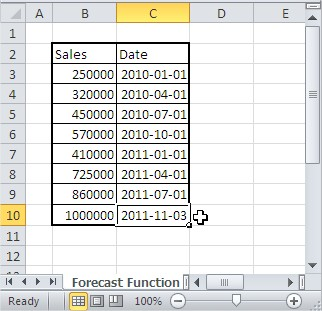The result is 2011-11-03. Excel predicts that your company will have 1 million USD in sales on that day.

There are some basic examples. Of course, there are also many ways to use the forecast function for business. The forecast function is easy to use and really powerful.

## Tip in case of errors:

If the x argument  is nonnumeric, the FORECAST function returns the #VALUE! error.

If known_y’s and known_x’s are empty or contain a different number of data points, the FORECAST function returns the #N/A error.

If the variance of the known_x argument is zero, the function FORECAST returns the #DIV/0! error.

## Key Takeaways

• The FORECAST function is a powerful tool that can be used to predict future values based on historical data.
• The FORECAST function has three required arguments: x, known_y’s, and known_x’s.
• The FORECAST function can be used to predict a wide variety of values, including sales, net income, and dates.

## FAQ

• Q: What is the difference between the FORECAST function and the TREND function?
• A: The FORECAST function and the TREND function are both used to predict future values based on historical data. However, the FORECAST function is a more general-purpose function, while the TREND function is specifically designed for predicting linear trends.
• Q: What are some common errors that people make when using the FORECAST function?
• A: Some common errors that people make when using the FORECAST function include: * Using a non-numeric value for the x argument. * Using an empty range for the known_y’s or known_x’s arguments. * Using a range with a different number of data points for the known_y’s and known_x’s arguments. * Using a range with a variance of zero for the known_x’s argument.